# Lebesgue space

(diff) ← Older revision | Latest revision (diff) | Newer revision → (diff)
A measure space(whereis a set,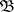is a-algebra of subsets of, called measurable sets, andis a measure defined on the measurable sets), isomorphic to the "standard model" , consisting of an intervaland an at most countable set of points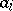(in "extreme" cases this "model" may consists of just the intervalor of just the points) endowed with the following measure: onone takes the usual Lebesgue measure, and to each of the pointsone ascribes a measure; the measure is assumed to be normalized, that is,. The "isomorphism" can be understood here in the strict sense or modulo; one obtains, respectively, a narrower or wider version of the concept of a Lebesgue space (in the latter case one can talk about a Lebesgue space modulo). One can give a definition of a Lebesgue space in terms of "intrinsic" properties of the measure space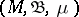(see ).
A Lebesgue space is the most frequently occurring type of space with a normalized measure, since any complete separable metric space with a normalized measure (defined on its Borel subsets and then completed in the usual way) is a Lebesgue space. Apart from properties common to all measure spaces, a Lebesgue space has a number of specific "good" properties. For example, any automorphism of a Boolean-algebra on a measure space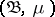is generated by some automorphism of a Lebesgue space. Under a number of natural operations, from a Lebesgue space one again obtains a Lebesgue space. Thus, a subsetof positive measure in a Lebesgue spaceis itself a Lebesgue space (its measurable subsets are assumed to be those that are measurable in, and the measure is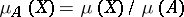); the direct product of finitely or countably many Lebesgue spaces is a Lebesgue space. Other properties of Lebesgue spaces are connected with measurable partitions (cf. Measurable decomposition).